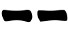# One Card

As for portability, nothing can stay on par with a single playing card. A regular playing card, however, would not offer much possibilities, so I devised a card design that allows casting I Ching hexagrams.
You can put it in your favourite I Ching book and having it immediately ready for when you need it.

The image below shows the front and the back side of the card:

You can download an hires version (300dpi) of the two images and print them on photographic paper, glue them together and, possibly, laminate. With little effort, this card will serve you well for long time.  By chance the images also happen to be of the right size to be printed as bridge-sized custom cards on MPC (with which I have no relationship).

The card can be used to obtain lines with different probabilities.

#### Three coins probabilities

You need two operations for each line. Proceed as follows:
1. Without looking, turn and rotate the card in your hand so that you no longer know which face shows nor its orientation.
2. When you feel the time is right, look at the card.
• If the red tiger shows up, draw;
• If the red dragon shows up, draw;
3. Repeat step 1;
4. Look at the upper left corner. If you see a red dot, it's a moving line.
5. Repeat steps 1-4 other five times and draw the line from the bottom to the top of the hexagram.

Probabilities
The firt time we only cosider which face shows up (front or back) each with a probability of  1/2 . The second time we look for the only corner, among the four, that has a red dot. Hence the probabilities are the same of the three coins method:
Prob(6) = Prob(9) = 1/2 * 1/4 = 1/8 = 12.5%
Prob(8) = Prob(7) = 1/2 * 3/4 = 3/8 = 37.5%
Prob(yin) = Prob(yang) = 1/2

#### Yarrow Stalks probabilities

You need two operations for each line. Proceed as follows:
1. Without looking, turn and rotate the card in your hand so that you no longer know which face shows nor its orientation.
2. When you feel the time is right, look at the upper left corner of the card and write down the number of black dots (either 3 or 4) you see;
3. Repeat step 1;
4. When you feel the time is right, look at the upper left corner of the card and write down the number of dots, regardless the color, (either 3, 4 or 5) you see;
5. Sum up the numbers and draw the line according the following table:
 6 7 8 96. Repeat steps 1-5 other five times and draw the lines from the bottom to the top of the hexagram.
Probabilities
There are four possible orientation for a card (front+up, front+down, back+up, back+down) and the four possible "upper left corner". The card is marked so that the first time we can get four possible outcomes: 3,4,4,4 while the second time we can get 3,4,4,5. Summing up as shown in the following table:

gives the same probabilities of the yarrow stalks method:
Prob(6) = 1/16 = 6.25%
Prob(8) = 7/16 = 43.75%
Prob(7) = 5/16 = 31.25%
Prob(9) = 3/16 = 18.75%
Prob(yin) = Prob(yang) = 1/2

#### Equal Probabilities

Proceed as follows:
1. Without looking, turn and rotate the card in your hand so that you no longer know which face shows nor its orientation.
2. When you feel the time is right, look at the card.
• If the red tiger shows up, draw;
• If the red dragon shows up, draw;
3. If the card is upside down (i.e. the flame is pointing downward), it's a moving line.
4. Repeat steps 1-3 other five times and draw the lines from the bottom to the top of the hexagram
Probabilities
Each line has the same probability to be drawn:
Prob(6) = Prob(back+down) = 1/4 = 25%
Prob(8) = Prob(back+up)     = 1/4 = 25%
Prob(7) = Prob(fron+up)       = 1/4 = 25%
Prob(9) = Prob(front+down) = 1/4 = 25%
Prob(yin) = Prob(yang) = 1/2

Image on the card taken from the free "Clipart Panda" site.